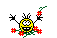###Author Topic: Anyone got code for a 3D(or flat) Hexagon?  (Read 555 times)

####JohnnyB

• Mc. Print
•• Posts: 37##### Anyone got code for a 3D(or flat) Hexagon?
« on: 2019-Aug-13 »
Just askin####SnooPI##### Re: Anyone got code for a 3D(or flat) Hexagon?
« Reply #1 on: 2019-Oct-14 »
A 3D hexagon (or hexagonal prism) is just a particular cylinder.
If you use this function with slices=6, you normally get a hexagonal prism (I have not tested).

CreateCylinder(0,  16, 16, 16,  6, 1,  RGB(255, 0, 0))

Code: (glbasic) [Select]
`FUNCTION CreateCylinder: num, baseRadius, topRadius, height, slices, stacks, colLOCAL da, r, dr, dz, x, y, z, i, jLOCAL x1, y1, x2, y2 da = 360 / slices dr = (topRadius-baseRadius) / stacks dz = height / stacks X_AUTONORMALS 2 X_OBJSTART num FOR i=0 TO slices-1 x1 = COS(i*da) y1 = SIN(i*da) x2 = COS((i+1)*da) y2 = SIN((i+1)*da) z = 0 r = baseRadius // Bottom lid IF baseRadius>0 X_OBJADDVERTEX 0, 0, 0,  .5, .5, col X_OBJADDVERTEX x1*r, y1*r, 0, x1/2+.5, y1/2+.5, col X_OBJADDVERTEX x2*r, y2*r, 0, x2/2+.5, y2/2+.5, col X_OBJNEWGROUP ENDIF // side FOR j=0 TO stacks X_OBJADDVERTEX x2*r, y2*r, z, (i+1)/slices,  j/stacks, col X_OBJADDVERTEX x1*r, y1*r, z,  i   /slices,  j/stacks, col z = z+dz r = r+dr NEXT // Top lid IF topRadius>0 X_OBJNEWGROUP X_OBJADDVERTEX x2*r, y2*r, height, x2/2+.5, y2/2+.5, col X_OBJADDVERTEX x1*r, y1*r, height, x1/2+.5, y1/2+.5, col X_OBJADDVERTEX 0, 0, height,  .5, .5, col ENDIF X_OBJNEWGROUP NEXT X_OBJENDENDFUNCTION`